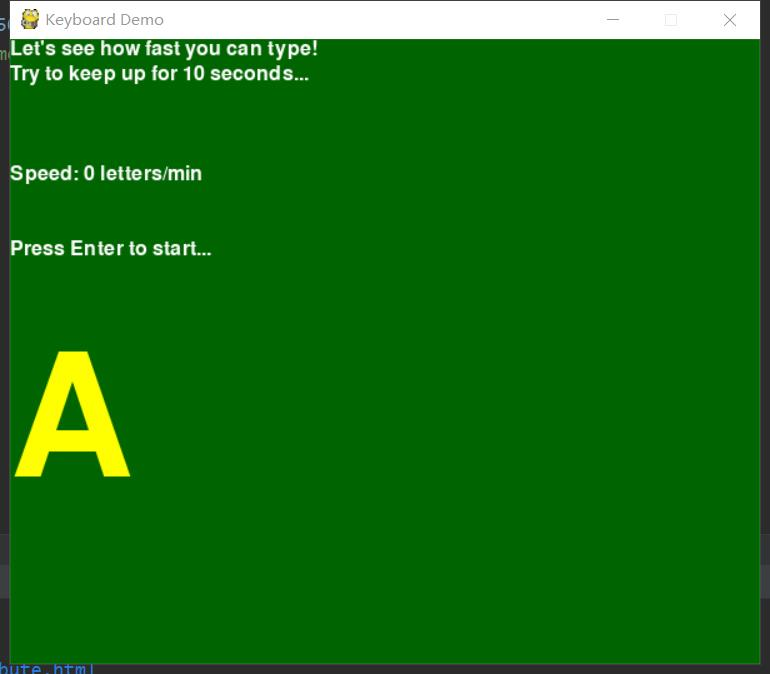# 20193427 2019-2020-2 《Python程序设计》实验四报告

## 1.实验内容

Python综合应用：爬虫、数据处理、可视化、机器学习、神经网络、游戏、网络安全等

import sys
import random
import time
import pygame
from pygame.locals import *

def print_text(font, x, y, text, color=(255, 255, 255)):
imgText = font.render(text, True, color)
screen.blit(imgText, (x, y))

pygame.init()
screen = pygame.display.set_mode((600, 500))
pygame.display.set_caption('Keyboard Demo')
font1 = pygame.font.Font(None, 24)
font2 = pygame.font.Font(None, 200)
white = (255, 255, 255)
yellow = (255, 255, 0)

key_flag = False
correct_answer = 97 # a
seconds = 11
score = 0
speed = 0
clock_start = 0
game_over = True

while True:
for event in pygame.event.get():
if event.type == QUIT:
sys.exit()
elif event.type == KEYDOWN:
key_flag = True
elif event.type == KEYUP:
key_flag = False
keys = pygame.key.get_pressed()
if keys[K_ESCAPE]:
sys.exit()
if keys[K_RETURN]:
if game_over:
game_over = False
clock_start = time.time()
score = 0
seconds = 11
speed = 0
clock = clock_start

if not game_over:
current = time.time() - clock_start
if seconds < current:
game_over = True
else:
if keys[correct_answer]:
correct_answer = random.randint(97, 122)
clock_start = time.time()
score += 1
speed = 60 * score / (clock_start - clock)

screen.fill((0, 100, 0))
print_text(font1, 0, 0, "Let's see how fast you can type!")
print_text(font1, 0, 20, "Try to keep up for 10 seconds...")

if key_flag:
print_text(font1, 500, 0, "<key>")

if not game_over:
print_text(font1, 0, 80, "Time: " + str(int(seconds-current)))

print_text(font1, 0, 100, "Speed: " + str(int(speed)) + " letters/min")

if game_over:
print_text(font1, 0, 160, "Press Enter to start...")

print_text(font2, 0, 240, chr(correct_answer-32), yellow)

pygame.display.update()


## 运行结果：## 3. 实验过程中遇到的问题和解决过程

• 问题1：在运行过程中安装库出现了问题
• 问题1解决方案：通过百度找帖子，询问同学，最后找到了解决的方法
• 问题2：有很多地方不是很熟练，会出现一些小错误而影响整体代码
• 问题2解决方案：通过观看视屏及笔记来复习所学的东西
• 问题三：刚开始不太清楚原理，无从下手
• 问题三解决方案：通过查询百度还有参考《Python游戏编程入门》一书解决了问题

## 其他（感悟、思考等）

• 通过这次实验我提高了自身的自主学习能力，能有效地利用周围的资源来提高自己，比如网络技术贴、身边的同学等等，在遇到一个问题首先先自己去思考、解决，而不是求助于人；
• 学过的知识要及时复习巩固，就像这次的实验很多内容都有或多或少的忘记，掌握的不是很熟练；
• 在编程过程中要更加细致小心，经常一个小小的细节，比如说符号或者缩进量就可能会导致整个程序的错误。

《python编程从入门到实践》
《Python游戏编程入门》

## 课程总结

通过十二周的python学习，使我对这门语言有了基本的认识，当然，我也学会了基本的操作。刚开始学习时，因为人们对他的一些固有的态度，


## 建议与意见

posted @ 2020-06-14 21:47  杜肚肚  阅读(141)  评论(0编辑  收藏